# Section A.3. ARITHMETIC OPERATIONS OF COMPLEX NUMBERS

### A 3 ARITHMETIC OPERATIONS OF COMPLEX NUMBERS

A.3.1 Addition and Subtraction of Complex Numbers

Which of the above forms for C in Eq. (A-1) is the best to use? It depends on the arithmetic operation we want to perform. For example, if we're adding two complex numbers, the rectangular form in Eq. (A-1) is the easiest to use. The addition of two complex numbers, C1 = R1 + jI1 and C2 = R2 + jI2, is merely the sum of the real parts plus j times the sum of the imaginary parts as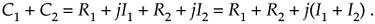Figure A-3 is a graphical depiction of the sum of two complex numbers using the concept of phasors. Here the sum phasor C1 + C2 in Figure A-3(a) is the new phasor from the beginning of phasor C1 to the end of phasor C2 in Figure A-3(b). Remember, the Rs and the Is can be either positive or negative numbers. Subtracting one complex number from the other is straightforward as long as we find the differences between the two real parts and the two imaginary parts separately. Thus

Equation A-11Figure A-3. Geometrical representation of the sum of two complex numbers.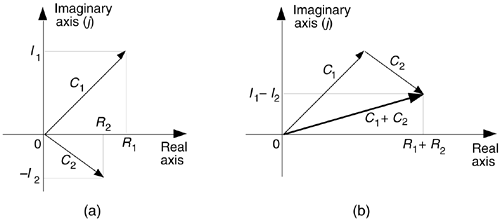An example of complex number addition is discussed in Section 11.3, where we covered the topic of averaging fast Fourier transform outputs.

A.3.2 Multiplication of Complex Numbers

We can use the rectangular form to multiply two complex numbers as

Equation A-12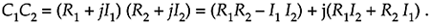However, if we represent the two complex numbers in exponential form, their product takes the simpler form

Equation A-13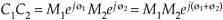because multiplication results in the addition of the exponents.

As a special case of multiplication of two complex numbers, scaling is multiplying a complex number by another complex number whose imaginary part is zero. We can use the rectangular or exponential forms with equal ease as follows:

Equation A-14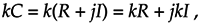or in exponential form,

Equation A-15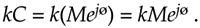A.3.3 Conjugation of a Complex Number

The complex conjugate of a complex number is obtained merely by changing the sign of the number's imaginary part. So, if we denote C* as the complex conjugate of the number C = R + jI = Mejø, then C* is expressed as

Equation A-16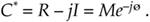There are two characteristics of conjugates that occasionally come in handy. First, the conjugate of a product is equal to the product of the conjugates. That is, if C = C1C2, then from Eq. (A-13),

Equation A-17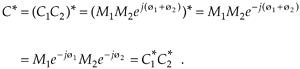Second, the product of a complex number and its conjugate is the complex number's magnitude squared. It's easy to show this in exponential form as

Equation A-18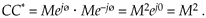(This property is often used in digital signal processing to determine the relative power of a complex sinusoidal phasor represented by Mejwt.)

A.3.4 Division of Complex Numbers

The division of two complex numbers is also convenient using the exponential and magnitude and angle forms, such as

Equation A-19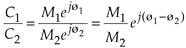and

Equation A-19'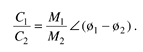Although not nearly so handy, we can perform complex division in rectangular notation by multiplying the numerator and the denominator by the complex conjugate of the denominator as

Equation A-20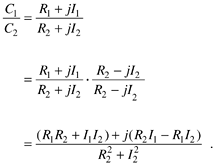A.3.5 Inverse of a Complex Number

A special form of division is the inverse, or reciprocal, of a complex number. If C = Mejø, its inverse is given by

Equation A-21In rectangular form, the inverse of C = R + jI is given by

Equation A-22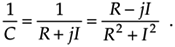We get Eq. (A-22) by substituting R1 = 1, I1 = 0, R2 = R, and I2 = I in Eq. (A-20).

A.3.6 Complex Numbers Raised to a Power

Raising a complex number to some power is easily done in the exponential form. If C = Mejø , then

Equation A-23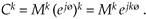For example, if C = 3ej125°, then C cubed is

Equation A-24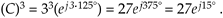We conclude this appendix with four complex arithmetic operations that are not very common in digital signal processing—but you may need them sometime.

A.3.7 Roots of a Complex Number

The kth root of a complex number C is the number that, multiplied by itself k times, results in C. The exponential form of C is the best way to explore this process. When a complex number is represented by C = Mejø, remember that it can also be represented by

Equation A-25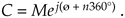In this case, the variable ø in Eq. (A-25) is in degrees. There are k distinct roots when we're finding the kth root of C. By distinct, we mean roots whose exponents are less than 360°. We find those roots by using the following:

Equation A-26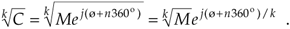Next, we assign the values 0, 1, 2, 3, . . ., k–1 to n in Eq. (A-26) to get the k roots of C. OK, we need an example here! Let's say we're looking for the cube (third) root of C = 125ej(75°). We proceed as follows:

Equation A-27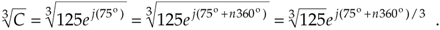Next we assign the values n = 0, n = 1, and n = 2 to Eq. (A-27) to get the three roots of C. So the three distinct roots areandA.3.8 Natural Logarithms of a Complex Number

Taking the natural logarithm of a complex number C = Mejø is straightforward using exponential notation; that is

Equation A-28where 0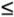ø < 2p . By way of example, if C = 12ejp/4, the natural logarithm of C is

Equation A-29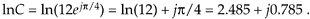This means that e(2.485 + j0.785) = e2.485 · ej0.785 = 12ejp/4.

A.3.9 Logarithm to the Base 10 of a Complex Number

We can calculate the base 10 logarithm of the complex number C = Mejø using

Equation A-30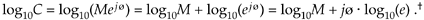Of course e is the irrational number, approximately equal to 2.71828, whose log to the base 10 is approximately 0.43429. Keeping this in mind, we can simplify Eq. (A-30) as

Equation A-31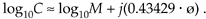Repeating the above example with C = 12ejp/4 and using the Eq. (A-31) approximation, the base 10 logarithm of C is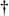For the second term of the result in Eq. (A-30) we used loga(xn) = n·logax according to the law of logarithms.

Equation A-32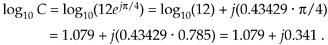The result from Eq. (A-32) means that

Equation A-33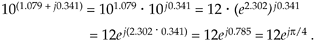A.3.10 Log to the Base 10 of a Complex Number Using Natural Logarithms

Unfortunately, some software mathematics packages have no base 10 logarithmic function and can calculate only natural logarithms. In this situation, we just use

Equation A-34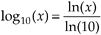to calculate the base 10 logarithm of x. Using this change of base formula, we can find the base 10 logarithm of a complex number C = Mejø; that is,

Equation A-35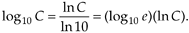Because log10(e) is approximately equal to 0.43429, we use Eq. (A-35) to state that

Equation A-36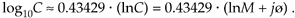Repeating, again, the example above of C = 12ejp/4, the Eq. (A-36) approximation allows us to take the base 10 logarithm of C using natural logs as

Equation A-37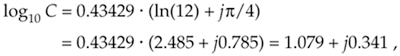giving us the same result as Eq. (A-32).

URL http://proquest.safaribooksonline.com/0131089897/app01lev1sec3AmazonPrev don't be afraid of buying books Next